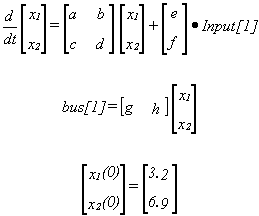• Introduction
• What is in this manual
• What is Caspoc
• User interface
• Introduction
• Starting
• Simulation
• Editing
• Viewing and printing
• Getting Started
• Basic editing
• Simulation in the time domain
• Basic User Interface Topics
• Editing
• Simulation
• Viewing
• Library
• Reports
• Project management
• Circuit and Block Diagram Components
• Introduction
• Cscript and user defined functions
• Component parameters
• Modeling Topics
• Introduction
• Power Electronics
• Semiconductors
• Electrical Machines
• Electrical drives
• Power Systems
• Mechanical Systems
• Thermal Systems
• Magnetic Circuits
• Green Energy
• Coupling to FEM
• Experimenter
• Analog hardware description language
• Embedded C code Export
• Coupling to Spice
• Small Signal Analysis
• Matlab coupling
• Tips and tricks
• Appendices

## MODELING LANGUAGE MISCELLANEOUS

Various topics of interest are explained with the use of the Pascal programming language. They also apply to other programming languages.

Borland Delphi
The modeling language Pascal can be compiled to a DLL file using the Borland Delphi 3.0 program.

### Local variables.

In the modeling language you can define your own variables, however they are defined local. This means that whenever a function in the modeling language is entered the local variables don't have a defined value. Leaving the function in the modeling language, the local defined variables loose their value.
 Good! Wrong!  VAR  x1,x2:real; BEGIN CASE procedure_number OF    1:BEGIN      x1 := bus;      bus:=x1+1;      END;    2:BEGIN      x2 := bus*2;      bus := x2;      END;  END;   VAR  x1,x2:real; BEGIN CASE procedure_number OF    1:BEGIN      x1 := bus +1;      END;    2:BEGIN      x2 := x1*2;      bus := x2;      END; END; 
The function in the modeling language are executed more than once per time step dt, because of the internal algorithms of CASPOC. However you can build a counter like:
 bus := bus+1;
It also enables you to sample data in the bus variables, by reading a sampled value in for example bus. Using bus number 99 as a trigger signal for sampling, you can shift the data on your bus. If you use bus till bus[n] for sampling, your code would look like :
  if bus>1 then     for i:=n downto 2 do       bus[i]:=bus[i-1];  bus:=NewSample; 

### Runtime errors

When you define Pascal code and are linking it to CASPOC, a runtime error during simulation can occur.
For example you define a bus variable, which doesn�t exists or you divide by zero.
CASPOC can't handle these errors. Borland Delphi causes the errors, which will occur.
The most common errors produced are:
-range error bus[i] i<=0 or i>=300
-divide error  1/0
-overflow  1e20*1e20*1e20
-not initialized variables
Remember that according to the calculation sequence first the system blocks and Pascal code are analyzed before the circuit is initialized.

### Differential equations

To define differential equations you can use the arrays x[i] and dxdt[i]. The differential equations have to be implemented as a set of first order differential equations. For example the state space equationscan be implemented as
 dxdt:=a*x+b*x+e*input; dxdt:=c*x+b*x+f*input; bus:=g*x+h*x;  Initialization of the state variables x[i] can be performed if p equals 0.
 CASE p OF   0: BEGIN      x0:=3.2;      x0:=6.9;      END;   1: BEGIN      ........  The same integration method used for the block diagram method is applied for the integration method in the Modeling Language.
 VAR i : INTEGER; CASE p OF   0: BEGIN      bus:=3.7;      bus:=sin(PI/3);      FOR i:=1 TO 30 DO           bus[20+i]:=i*4;      END;   1: BEGIN      ........